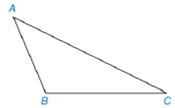Chapter 7.1, Problem 6E### Elementary Geometry for College St...

6th Edition
Daniel C. Alexander + 1 other
ISBN: 9781285195698

#### Solutions

Chapter
Section### Elementary Geometry for College St...

6th Edition
Daniel C. Alexander + 1 other
ISBN: 9781285195698
Textbook Problem
1 views

# In Exercises 3 to 8, use the drawing provided.Given: Obtuse ∆ A B C Construct: The perpendicular bisector of A C -

To determine

To construct:

The perpendicular bisector of AC- for the obtuse ABC as shown in the given figure.

Explanation

Definition:

Perpendicular bisector of a triangle is a line passing through the midpoint of the particular side of the triangle.

Calculation:

The given obtuse ABC is shown below:

To construct a bisector we follow the below procedure.

Step 1: Using the compass, take a length of two-third of the length of AC and take A as centre, draw an arc inside of the triangle.

Step 2: Similarly, take C as centre, draw an arc in the same side of arc drawn earlier to obtain a point D

### Still sussing out bartleby?

Check out a sample textbook solution.

See a sample solution

#### The Solution to Your Study Problems

Bartleby provides explanations to thousands of textbook problems written by our experts, many with advanced degrees!

Get Started

#### Evaluate the expression sin Exercises 116. (23)2

Finite Mathematics and Applied Calculus (MindTap Course List)

#### Let f(x) = 1x+1 and g(x) = x2 + 1. Find the rules for (a) f + g, (b) fg (c) f t, and (d) g f.

Applied Calculus for the Managerial, Life, and Social Sciences: A Brief Approach

#### Which curve is simple but not closed?

Study Guide for Stewart's Multivariable Calculus, 8th